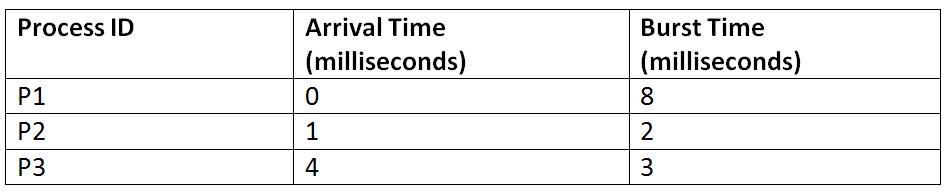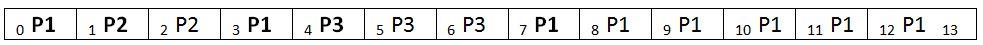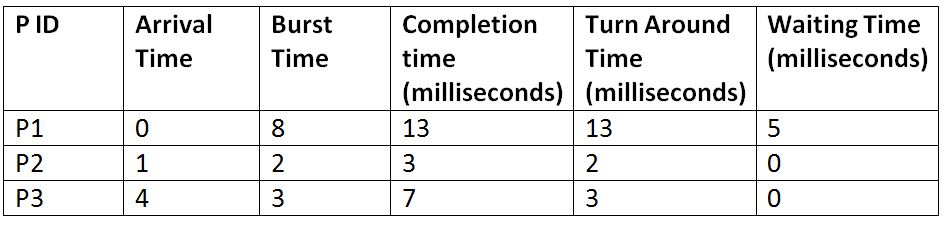# SRTF: Shortest Remaining Time First Scheduling Algorithm

This article is about the SRTF Scheduling Algorithm in Operating System. In this article, we are going to study about the SRTF, i.e. Shortest Remaining Time First Scheduling Algorithm. We will first define it briefly and will then illustrate it with the help of an example for understanding it better.
Submitted by Monika Sharma, on June 23, 2019

SRTF, Which Stands for Shortest Remaining Time First is a scheduling algorithm used in Operating Systems, which can also be called as the preemptive version of the SJF scheduling algorithm. The process which has the least processing time remaining is executed first. As it is a preemptive type of schedule, it is claimed to be better than SJF scheduling Algorithm.

Let's understand this with the help of an example. Suppose we have the following 3 processes with process ID's P1, P2, and P3 and they arrive into the CPU in the following manner:Gant Chart:Explanation:

• At the 0th unit of the CPU, we have only process P1, so it gets executed for the 1-time unit.
• At the 1st unit of the CPU, the Process P2 also arrives. Now, the P1 needs 7 more units more to be executed, and P2 needs only 2 units. So, P2 is executed by preempting P1.
• P2 gets completed at time unit 3, and unit now no new process has arrived. So, after the completion of P2, again P1 is sent for execution.
• Now, P1 has been executed for one unit only, and we have an arrival of new process P3 at time unit 4. Now, the P1 needs 6-time units more and P3 needs only 3-time units. So, P3 is executed by preempting P1.
• P1 gets completed at time unit 7, and after that, we have the arrival of no other process. So again, P1 is sent for execution, and it gets completed at 13th unit.```    Total Turn Around Time = 13 + 2 + 3
= 18 milliseconds
Average Turn Around Time= Total Turn Around Time / Total No. of Processes
= 18 / 3
= 6 milliseconds

Total Waiting Time = 5 + 0 + 0
= 5 milliseconds
Average Waiting Time = Total Waiting Time / Total No. of Processes
= 5 / 3
= 1.67 milliseconds
```

What's New

Top Interview Coding Problems/Challenges!

Languages: » C » C++ » C++ STL » Java » Data Structure » C#.Net » Android » Kotlin » SQL
Web Technologies: » PHP » Python » JavaScript » CSS » Ajax » Node.js » Web programming/HTML
Solved programs: » C » C++ » DS » Java » C#
Aptitude que. & ans.: » C » C++ » Java » DBMS
Interview que. & ans.: » C » Embedded C » Java » SEO » HR
CS Subjects: » CS Basics » O.S. » Networks » DBMS » Embedded Systems » Cloud Computing
» Machine learning » CS Organizations » Linux » DOS
More: » Articles » Puzzles » News/Updates